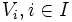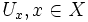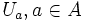# Compact implies feebly compact

This article gives the statement and possibly, proof, of an implication relation between two topological space properties. That is, it states that every topological space satisfying the first topological space property (i.e., compact space) must also satisfy the second topological space property (i.e., feebly compact space)
View all topological space property implications | View all topological space property non-implications
Get more facts about compact space|Get more facts about feebly compact space

## Definitions used

### Compact space

Further information: Compact space

A topological space is termed compact if every open cover of the space has a finite subcover.

### Feebly compact space

A topological space is termed feebly compact if every locally finite collection of nonempty open subsets is finite.

## Proof

Given: A compact space$X$, a locally finite collection of nonempty open subsets$V_i, i \in I$ of$X$.

To prove:$I$ is finite.

Proof:

1. There exists a point-indexed open cover$U_x, x \in X$ of$X$ such that each$U_x$ intersects finitely many$V_i$s: For each point$x \in X$, we can find an open subset$U_x$ that works by the definition of locally finite. Putting these together, there exists a point-indexed open cover.
2. There exists a finite subset$A$ of$X$ such that the$U_a, a \in A$, cover$X$: This follows from the previous step and the definition of compactness.
3. The size of$I$ is finite: Every$V_i,i \in I$, is a nonempty open subset of$X$, hence it intersects at least one of the$U_a$s. Thus, to count the$V_i$s, it suffices to count the$V_i$s that intersect at least one$U_a$. For each$U_a$, the number of$V_i$s intersecting it is finite, and there are finitely many$a$s in$A$, so the total number is finite.